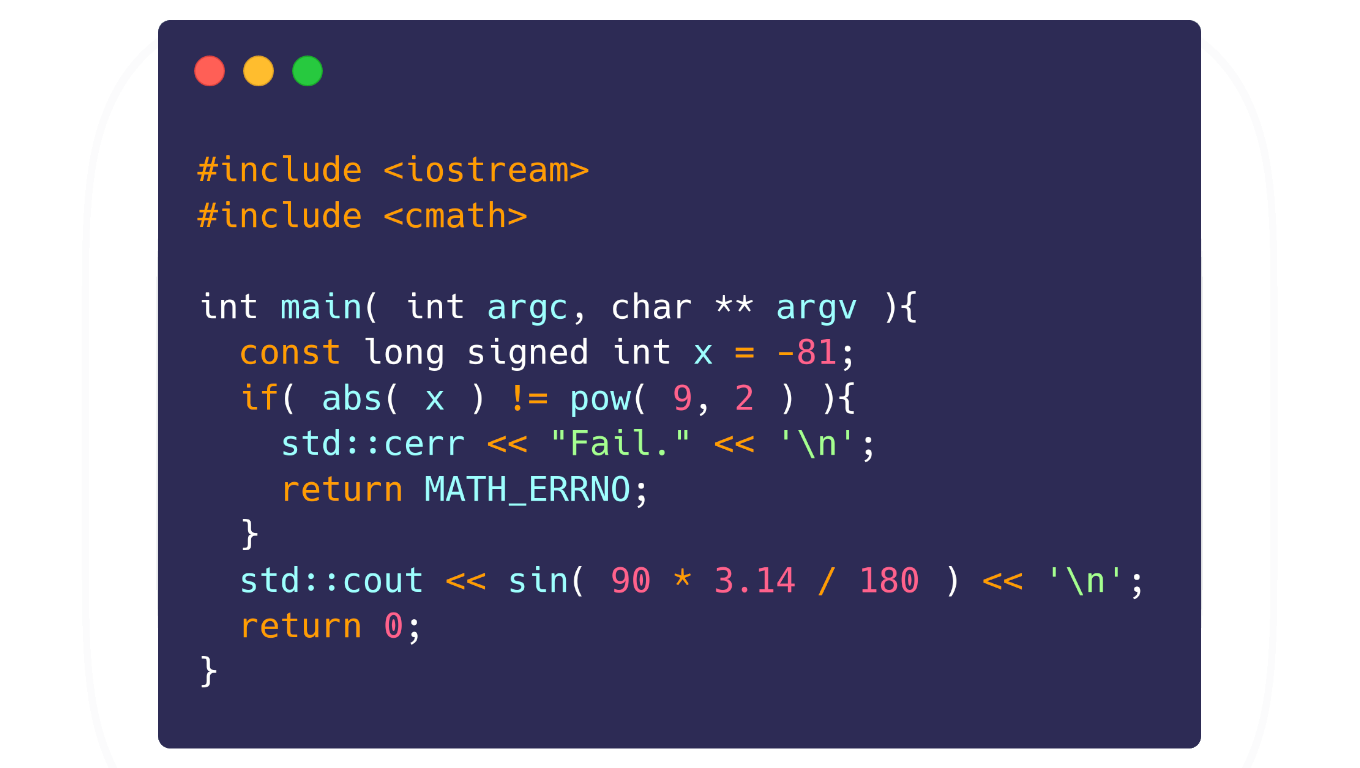# Working with cmath in C++

### Making Mathematical Calculations Simpler to Get Results.The `cmath` library in C++ is the same as math.h in C and it supports a large number of useful math functions, the complete list of all functions you can see here.

To use them, just include it in the header: `#include <cmath>`

In this cpp::daily of today we will see description and how to use some of them.

• `abs( int )` - Informs the absolute number:
• `pow( double, double )` - Is the power of two numbers:

• `sin( double * pi / 180 )` - tells the sine of an angle;
• `cos( double * pi / 180 )` - gives the cosine of an angle;
• `tan( double * pi / 180 )` - tells the tangent of an angle; In this example we also include iomanip to use `std::setprecision( int )`
• We also use parameter via command line to get results at runtime
• We capture `argv` as a string and later convert it to a long double with `std::stold( std::string )`;
• Remembering that PI is an infinite number, therefore, we assign 13 significant digits (digits) for a greater precision of the result, as only 3.14 can have less accurate results.

Output:• `sqrt( double )` - Informs the square root of a number:
• For this example (mini program) in addition to using parameters, we also use colored outputs to make it stand out.

And among others like:

• `ceil( T )` - Rounds a number up: `ceil( 2.1f );` // 3 ;
• `cbrt( T )` - Informs the cubic root of a number: `cbrt( 27 );` // 3;
• `log( double )` - Calculates the logarithm.